xVolumetric flow rateEncyclopedia
The volumetric flow rate in fluid dynamics
Fluid dynamics
In physics, fluid dynamics is a sub-discipline of fluid mechanics that deals with fluid flow—the natural science of fluids in motion. It has several subdisciplines itself, including aerodynamics and hydrodynamics...

and hydrometry
Hydrometry
Hydrometry is the monitoring of the components of the hydrological cycle including rainfall, groundwater characteristics, as well as water quality and flow characteristics of surface waters...

, (also known as volume flow rate or rate of fluid flow) is the volume of fluid which passes through a given surface per unit time (for example cubic meters per second [m3 s-1] in SI
Si
Si, si, or SI may refer to :- Measurement, mathematics and science :* International System of Units , the modern international standard version of the metric system...

units, or cubic feet
Cubic foot
The cubic foot is an Imperial and US customary unit of volume, used in the United States and the United Kingdom. It is defined as the volume of a cube with sides of one foot in length.-Conversions:- Symbols :...

per second [cu ft/s]). It is usually represented by the symbol Q.

Volumetric flow rate should not be confused with volumetric flux
Volumetric flux
In fluid dynamics, the volumetric flux is the rate of volume flow across a unit area . The density of a particular property in a fluid's volume, multiplied with the volumetric flux of the fluid, thus defines the advective flux of that property...

, as defined by Darcy's law
Darcy's law
Darcy's law is a phenomenologically derived constitutive equation that describes the flow of a fluid through a porous medium. The law was formulated by Henry Darcy based on the results of experiments on the flow of water through beds of sand...

and represented by the symbol q, with units of m3/(m2 s), that is, m s-1. The integration of a flux over an area gives the volumetric flow rate.

Given an area
Area
Area is a quantity that expresses the extent of a two-dimensional surface or shape in the plane. Area can be understood as the amount of material with a given thickness that would be necessary to fashion a model of the shape, or the amount of paint necessary to cover the surface with a single coat...

A, and a fluid flowing through it with uniform velocity
Velocity
In physics, velocity is speed in a given direction. Speed describes only how fast an object is moving, whereas velocity gives both the speed and direction of the object's motion. To have a constant velocity, an object must have a constant speed and motion in a constant direction. Constant ...

C with an angle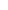away from the perpendicular
Perpendicular
In geometry, two lines or planes are considered perpendicular to each other if they form congruent adjacent angles . The term may be used as a noun or adjective...

direction to A, the flow rate is: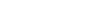.

In the special case where the flow is perpendicular to the area A, that is,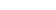, the volumetric flow rate is: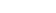.

The equation above is commonly referred to as the continuity equation (for one-dimensional incompressible flow
Incompressible flow
In fluid mechanics or more generally continuum mechanics, incompressible flow refers to flow in which the material density is constant within an infinitesimal volume that moves with the velocity of the fluid...

s). If the velocity of the fluid through the area is non-uniform (or if the area is non-planar) then the rate of fluid flow can be calculated by means of a surface integral
Surface integral
In mathematics, a surface integral is a definite integral taken over a surface ; it can be thought of as the double integral analog of the line integral...

: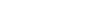where dS is a differential surface described by: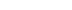with n the unit surface normal
Surface normal
A surface normal, or simply normal, to a flat surface is a vector that is perpendicular to that surface. A normal to a non-flat surface at a point P on the surface is a vector perpendicular to the tangent plane to that surface at P. The word "normal" is also used as an adjective: a line normal to a...

and dA the differential magnitude of the area.

If a surface S encloses a volume V, the divergence theorem
Divergence theorem
In vector calculus, the divergence theorem, also known as Gauss' theorem , Ostrogradsky's theorem , or Gauss–Ostrogradsky theorem is a result that relates the flow of a vector field through a surface to the behavior of the vector field inside the surface.More precisely, the divergence theorem...

states that the rate of fluid flow through the surface is the integral of the divergence
Divergence
In vector calculus, divergence is a vector operator that measures the magnitude of a vector field's source or sink at a given point, in terms of a signed scalar. More technically, the divergence represents the volume density of the outward flux of a vector field from an infinitesimal volume around...

of the velocity vector field
Vector field
In vector calculus, a vector field is an assignmentof a vector to each point in a subset of Euclidean space. A vector field in the plane for instance can be visualized as an arrow, with a given magnitude and direction, attached to each point in the plane...

C on that volume: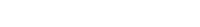.

• Air to cloth ratio
Air to cloth ratio
The air-to-cloth ratio is the volumetric flow rate of air flowing through a dust collector's inlet duct divided by the total cloth area in the filters...

• Darcy's law
Darcy's law
Darcy's law is a phenomenologically derived constitutive equation that describes the flow of a fluid through a porous medium. The law was formulated by Henry Darcy based on the results of experiments on the flow of water through beds of sand...

• Discharge (hydrology)
Discharge (hydrology)
In hydrology, discharge is the volume rate of water flow, including any suspended solids , dissolved chemical species and/or biologic material , which is transported through a given cross-sectional area...

• Flow measurement
Flow measurement
Flow measurement is the quantification of bulk fluid movement. Flow can be measured in a variety of ways.Positive-displacement flow meters acumulate a fixed volume of fluid and then count the number of times the volume is filled to measure flow...

• Flowmeter
• Flux
Flux
In the various subfields of physics, there exist two common usages of the term flux, both with rigorous mathematical frameworks.* In the study of transport phenomena , flux is defined as flow per unit area, where flow is the movement of some quantity per time...

(transport definition)
• Mass flow rate
Mass flow rate
Mass flow rate is the mass of substance which passes through a given surface per unit time. Its unit is mass divided by time, so kilogram per second in SI units, and slug per second or pound per second in US customary units...

• Orifice plate
Orifice plate
An orifice plate is a device used for measuring the volumetric flow rate. It uses the same principle as a Venturi nozzle, namely Bernoulli's principle which states that there is a relationship between the pressure of the fluid and the velocity of the fluid...

• Poiseuille's law
• Units of flow